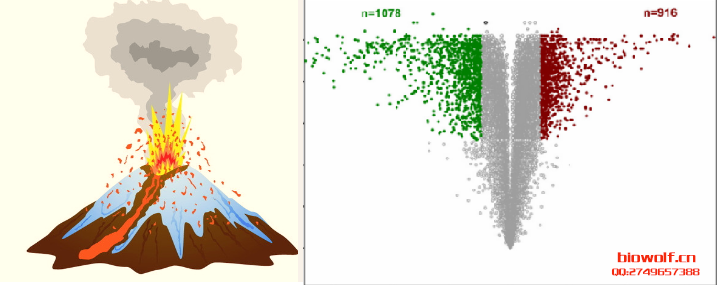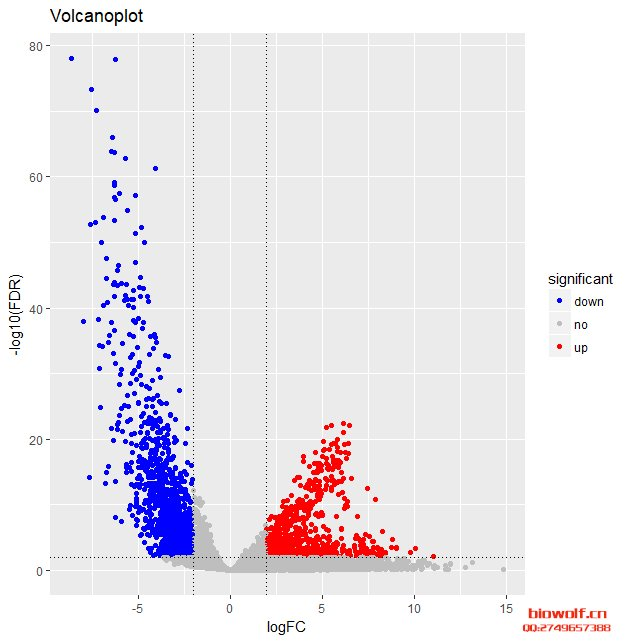### TCGA差异基因做火山图

TCGA数据库数据下载得到表达矩阵，用表达矩阵做差异分析，可以得到差异基因，以及差异基因相关的logFC和矫正后的P值，利用这两列数据可以画一个火山图，直观表示差异基因的上调下ggplot2是R语言的一个包，也是一个绘图系统，它吸收了R语言基础绘图及lattice绘图的优点，并应用图像图形学的理论来进行图像的绘制。

 ```setwd("D:\\FreeVideo\\99vol_plot") library(ggplot2) biowolf<-read.table(file="tcga_volcano_plot.txt",header=TRUE,row.names = 1) volcano<-ggplot(biowolf,aes(x=logFC,y=-log10(FDR))) volcano+geom_point(aes(color=significant)) +scale_color_manual(values=c("blue","grey","red")) +labs(title="Volcanoplot",x="logFC",y="-log10(FDR)") +geom_hline(yintercept=2,linetype=3) +geom_vline(xintercept=c(-2,2),linetype=3) ```BioWolf二维码生成器
------分隔线----------------------------